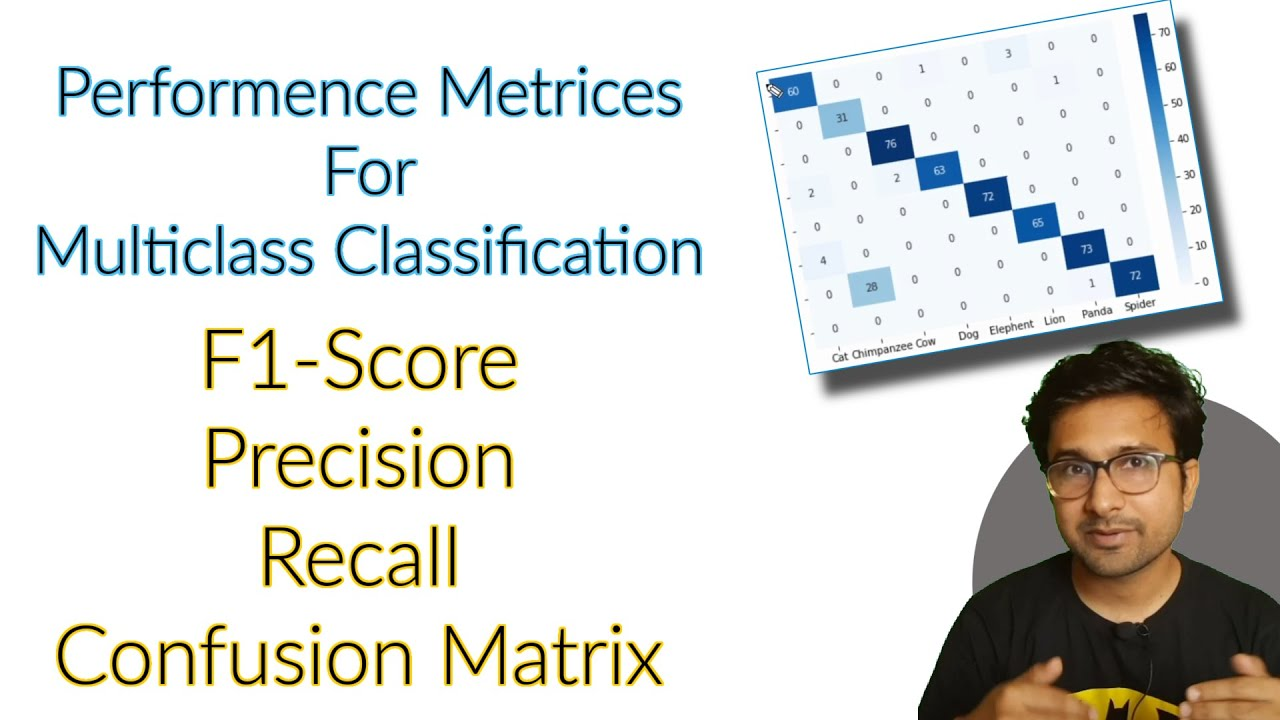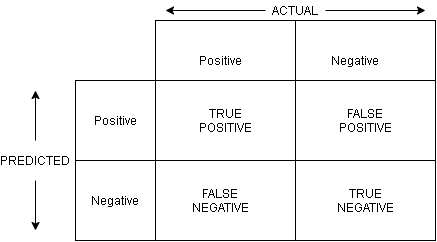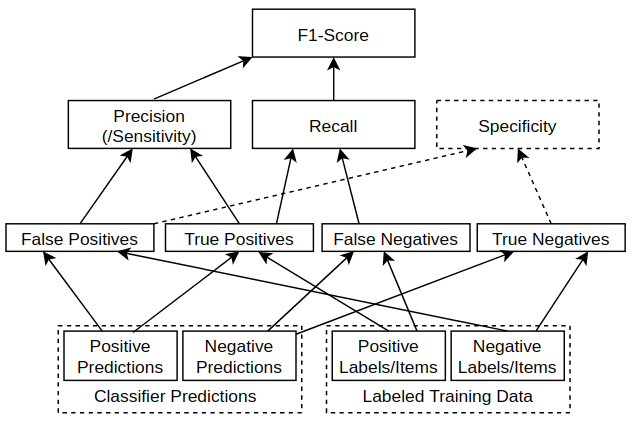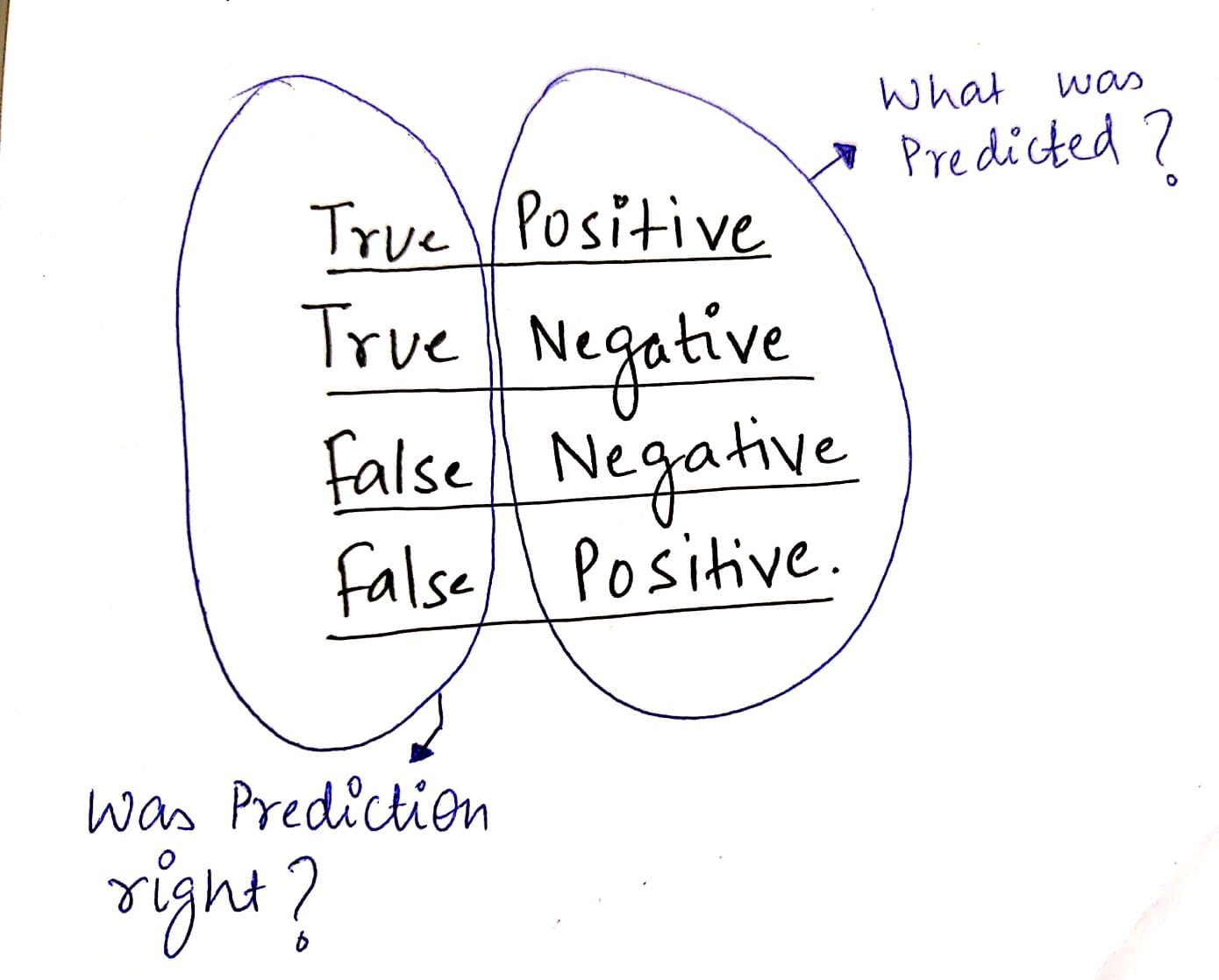1619593112

# Write your own function for Multiclass Classification Confusion Matrix, F1 Score, Precision, recall

In this video I have taken an example of multiclass image classification and explained how you can write your own function for calculating macro and micro accuracy precision-recall and f1-score with a confusion matrix. Here is the link for downloading the dataset I have used within this video

Chapters:
0:00​ Introduction
0:42​ dataset Explanation
3:01​ Dataset preparation
7:01​ Confusion matrix multiclass
18:25​ Overall accuracy precision-recall
21:00​ Macro and Micro precision recall

https://github.com/jakkcoder/dataset_…

#machine-learning #deep-learning

## Buddha Community1603436400

## Understanding Confusion Matrix, Precision-Recall, and F1-Score

As I was going over several notebooks in Kaggle the past few days, I couldn’t help but notice some notebooks titled _“Achieving 100% accuracy on dataset_name”, “Perfect 100% accuracy using algorithm_name”, _along with various other guides on how to achieve a 100% accuracy on every dataset you would come across. While some of these notebooks did a great job at building a generalized model for the dataset and delivering pretty good results, a majority of them were just overfitting on the data.

Overfitting_ is the production of an analysis that corresponds too closely or exactly to a particular set of data, and may therefore fail to fit additional data or predict future observations reliably — _Wikipedia

And the saddest part about all this? They weren’t even realizing that they were overfitting on the dataset while trying to achieve that golden number. Most of these notebooks were found on beginner-friendly datasets like the “Iris dataset” or “Titanic dataset” and it makes sense, right? Most of us while starting on the Machine Learning trajectory was only taught one thing: “Accuracy matters”. And while this is true, it matters only up to a certain extent. This is why I’ll be discussing some other performance metrics like Confusion Matrix, Precision-Recall, and F1-Score that you should consider using along with Accuracy while evaluating a Machine Learning model. Let’s get started.

## Confusion Matrix

_In the field of machine learning and specifically the problem of statistical classification, a confusion matrix, also known as an error matrix, is a specific table layout that allows visualization of the performance of an algorithm, typically a supervised learning one. — _WikipediaConfusion Matrix for a two-class classification problem (Image Source: Author)

To understand the confusion matrix let us consider a two-class classification problem with the two outcomes being “Positive” and “Negative”. Given a data point to predict, the model’s outcome will be any one of these two.

If we plot the predicted values against the ground truth (actual) values, we get a matrix with the following representative elements:

**True Positives (TP): **These are the data points whose actual outcomes were positive and the algorithm correctly identified it as positive.

**True Negatives (TN): **These are the data points whose actual outcomes were negative and the algorithm correctly identified it as negative.

**False Positives (FP): **These are the data points whose actual outcomes were negative but the algorithm incorrectly identified it as positive.

**False Negatives (FN): **These are the data points whose actual outcomes were positive but the algorithm incorrectly identified it as negative.

As you can guess, the goal of evaluating a model using the confusion matrix is to maximize the values of TP and TN and minimize the values of FP and FN.

#confusion-matrix #machine-learning #precision-recall #evaluation-metric #f1-score1604293620

## A Look at Precision, Recall, and F1-Score

Terminology of a specific domain is often difficult to start with. With a software engineering background, machine learning has many such terms that I find I need to remember to use the tools and read the articles.

Some basic terms are Precision, Recall, and F1-Score. These relate to getting a finer-grained idea of how well a classifier is doing, as opposed to just looking at overall accuracy. Writing an explanation forces me to think it through, and helps me remember the topic myself. That’s why I like to write these articles.

I am looking at a binary classifier in this article. The same concepts do apply more broadly, just require a bit more consideration on multi-class problems. But that is something to consider another time.

Before going into the details, an overview figure is always nice:Hierarchy of Metrics from raw measurements / labeled data to F1-Score. Image by Author.

On the first look, it is a bit of a messy web. No need to worry about the details for now, but we can look back at this during the following sections when explaining the details from the bottom up. The metrics form a hierarchy starting with the the _true/false negatives/positives _(at the bottom), and building up all the way to the _F1-score _to bind them all together. Lets build up from there.

## True/False Positives and Negatives

A binary classifier can be viewed as classifying instances as positive or negative:

• Positive: The instance is classified as a member of the class the classifier is trying to identify. For example, a classifier looking for cat photos would classify photos with cats as positive (when correct).
• Negative: The instance is classified as not being a member of the class we are trying to identify. For example, a classifier looking for cat photos should classify photos with dogs (and no cats) as negative.

#recall #f1-score #precision #data-science1619593112

## Write your own function for Multiclass Classification Confusion Matrix, F1 Score, Precision, recall

In this video I have taken an example of multiclass image classification and explained how you can write your own function for calculating macro and micro accuracy precision-recall and f1-score with a confusion matrix. Here is the link for downloading the dataset I have used within this video

Chapters:
0:00​ Introduction
0:42​ dataset Explanation
3:01​ Dataset preparation
7:01​ Confusion matrix multiclass
18:25​ Overall accuracy precision-recall
21:00​ Macro and Micro precision recall

https://github.com/jakkcoder/dataset_…

#machine-learning #deep-learning1620980580

## Recall Management Software

Initiate a product recall or stock withdrawal across all your locations simultaneously from Originscale recall management software in a matter of seconds.

#product recall #recall record management #recall management #recall software #manage recall #recall technology1597940460

## Confusion Matrix is no more confusing.

Before we switch into the topic, lets understand why we need to consider Confusion matrix and metrics ?

Metrics plays a major role in evaluating the performance of the model.

Metrics from Confusion Matrix.

• Confusion Matrix (Precision, Recall, F score, Accuracy)

Confusion Matrix is no more Confusing.

Consider a dataset has two classes say Class A and B. There may be two cases where your dataset is **Balanced **and Imbalanced. Balanced dataset means that, records for class A and B are balanced. Say Class A has 50% of data and class B has 50% of data or 55–45% of data. Imbalanced dataset has records of 90–10% of Class A and B or 80–20 and 70–30% of data.

Metrics to consider will be different for both Balanced and Imbalanced dataset.

Confusion Matrix comes with rows and columns of Actual and Predicted. The terminologies used are True Positive, True Negative, False positive, False Negative.

Lets split the words as True and positive separately.

Positive : Class A ; Negative : Not a Class A(Class B)

True : Predicted is right ; False : Predicted is wrong